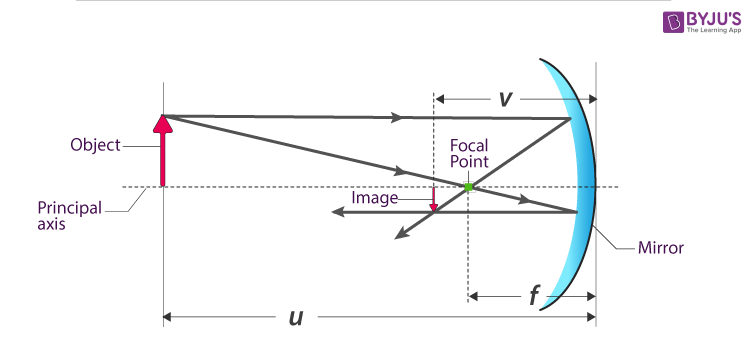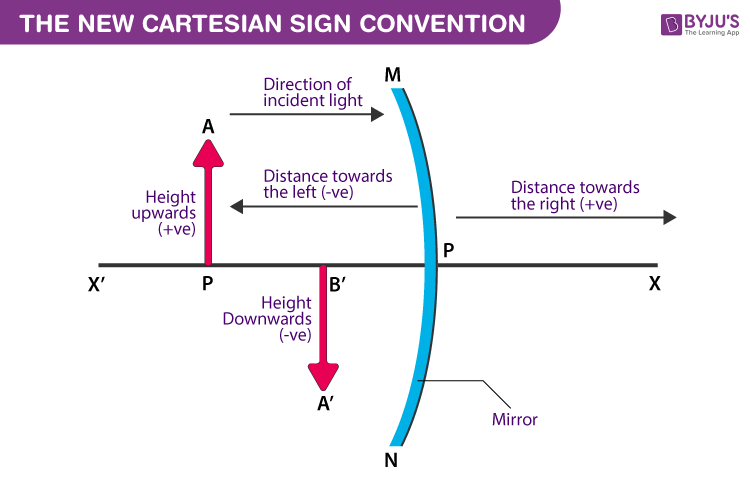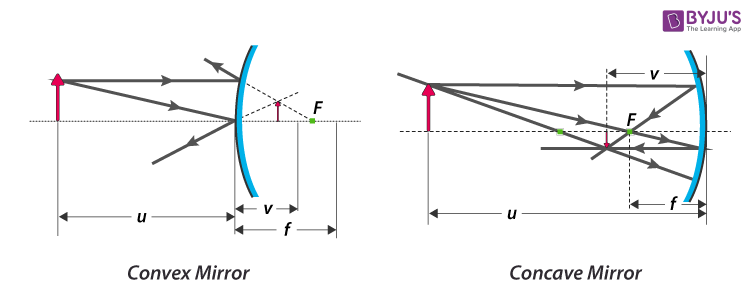Jet Set Go! All about Aeroplanes Jet Set Go! All about Aeroplanes

# Mirror Equation

## What Is Mirror Equation?

Mirror equation is an equation relating object distance and image distance with focal length. It is also known as the mirror formula.In a spherical mirror:

• The distance between the object and the pole of the mirror is called Object distance(u).
• The distance between the image and the pole of the mirror is called Image distance(v).
• The distance between the Principal focus and the pole of the mirror is called Focal Length(f).

In ray optics, The object distance, image distance, and Focal length are related as,

 $$\begin{array}{l}\frac{1}{v}+\frac{1}{u}=\frac{1}{f}\end{array}$$

Where,

• u is the Object distance
• v is the Image distance
• f is the Focal Length given by
$$\begin{array}{l}f=\frac{R}{2}\end{array}$$
• R is the radius of curvature of the spherical mirror

The above formula is valid under all situations for all types of (concave and convex) and for all object positions.

You may also want to check out these topics given below!

## Sign ConventionsNew Cartesian Sign Convention is used to avoid confusion in understanding the ray directions. Refer to the diagram for clear visualization.

• For the measurement of all the distances, the optical center of the lens is considered.
• When the distances are measured opposite to the direction of the incident light, they are considered to be negative.
• When the distances are measured in the same direction of the incident light, they are considered to be positive.
• When the heights are measured upwards and perpendicular to the principal axis, they are considered to be positive.
• When the heights are measured downwards and perpendicular to the principal axis, they are considered to be negative.

### Mirror Equation for Concave Mirror and Mirror Equation for a Convex MirrorThe mirror equation

$$\begin{array}{l}\frac{1}{v}+\frac{1}{u}=\frac{1}{f}\end{array}$$
holds good for concave mirrors as well as convex mirrors.

## Example of Mirror Equation

The radius of curvature of a convex mirror used for rearview on a car is 4.00 m. If the location of the bus is 6 meters from this mirror, find the position of the image formed.

Solution:

Given:

The radius of curvature (R)= +4.00 m

Object distance(u) = -6.00 m

Image distance(v) = ?

Formula used:

$$\begin{array}{l}f=\frac{R}{2}\end{array}$$
$$\begin{array}{l}\frac{1}{v}+\frac{1}{u}=\frac{1}{f}\end{array}$$

Calculation:

To calculate the Focal length of the given mirror, substitute the value of Radius of Curvature (R) in

$$\begin{array}{l}f=\frac{R}{2}\end{array}$$

We get-

$$\begin{array}{l}f=\frac{+4.00m}{2}=+2m\end{array}$$

Since,

$$\begin{array}{l}\frac{1}{v}+\frac{1}{u}=\frac{1}{f}\end{array}$$
we can re-arrange it as –

$$\begin{array}{l}\frac{1}{v}=\frac{1}{f}-\frac{1}{u}\end{array}$$

On substituting the values in the above equation, we get-

$$\begin{array}{l}\Rightarrow\frac{1}{v} =\frac{1}{+2.00}-\frac{1}{\left ( -6.00 \right )}\end{array}$$
$$\begin{array}{l}=\frac{1}{2.00}+\frac{1}{6.00}\end{array}$$
$$\begin{array}{l}\frac{6+2}{2\times 6}=\frac{8}{12}\end{array}$$
$$\begin{array}{l}v=\frac{12}{8}\end{array}$$

= 1.5 meter.

The image is 1.5 meters behind the mirror.

The mirror is a polished surface which reflects the incident light to form the image. Here reflected light will have a wavelength and many other physical properties almost the same as that of the incident light.

Test your Knowledge on Mirror Equation